Next: RELATED CONCEPTS Up: THE JENSEN INEQUALITY Previous: THE JENSEN INEQUALITY

## Examples of Jensen inequalities

The most familiar example of a Jensen inequality occurs when the weights are all equal to 1/N and the convex function is f(x) = x2. In this case the Jensen inequality gives the familiar result that the mean square exceeds the square of the mean: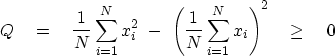(4)
In the other applications we will consider, the population consists of positive members, so the function f(p) need have a positive second derivative only for positive values of p. The function f(p)=1/p yields a Jensen inequality for the harmonic mean:(5)
A more important case is the geometric inequality. Here, and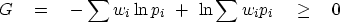(6)
The more familiar form of the geometric inequality results from exponentiation and a choice of weights equal to 1/N: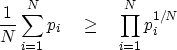(7)
In other words, the product of square roots of two values is smaller than half the sum of the values.

A Jensen inequality with an adjustable parameter is suggested by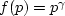:(8)
Whether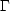is always positive or always negative depends upon the numerical value of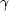.In practice we may see the dimensionless form, in which the ratio instead of the difference of the two terms is used.

A most important inequality in information theory and thermodynamics is the one based on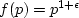,whereis a small positive number tending to zero. I call this the weak" inequality. With some calculation we will quickly arrive at the limit:(9)
Take logarithms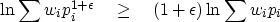(10)
Expand both sides in a Taylor series in powers ofusing(11)
The leading term is identical on both sides and can be canceled. Divide both sides byand go to the limit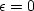,obtaining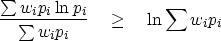(12)
We can now define a positive variable S' with or without a positive scaling factor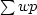: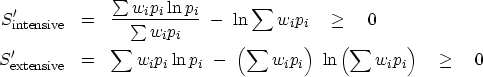(13) (14)
Seismograms often contain zeros and gaps. Notice that a single zero pi can upset the harmonic H or geometric G inequality, but a single zero has no horrible effect on S or.Next: RELATED CONCEPTS Up: THE JENSEN INEQUALITY Previous: THE JENSEN INEQUALITY
Stanford Exploration Project
10/21/1998